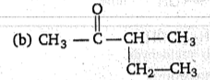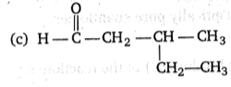• Subject:

...

• Topic:

...

Compound (A) C6H12O is optically active. Compound (A) give negative Tollens test and positive test with 2-4 dinitro phenyl hydrazine. Identify A.

(a) ${\mathrm{CH}}_{3}-\stackrel{\stackrel{\mathrm{O}}{\parallel }}{\mathrm{C}}-{\mathrm{CH}}_{2}-\underset{\stackrel{\mathrm{l}}{{\mathrm{CH}}_{3}}}{\mathrm{CH}}-{\mathrm{CH}}_{3}$(d) ${\mathrm{CH}}_{3}-\stackrel{\stackrel{\mathrm{O}}{\parallel }}{\mathrm{C}}-{\mathrm{CH}}_{2}-{\mathrm{CH}}_{2}-{\mathrm{CH}}_{2}-{\mathrm{CH}}_{3}$